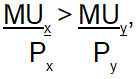Courses

# MCQs - Indifference Curve Commerce Notes | EduRev

## Commerce : MCQs - Indifference Curve Commerce Notes | EduRev

The document MCQs - Indifference Curve Commerce Notes | EduRev is a part of the Commerce Course Crash Course of Micro Economics -Class 12.
All you need of Commerce at this link: Commerce

Q.1 Hicks and Allen believed that utility
(a) Cannot be measured
(b) Cannot be expressed
(c)  Can be measured in cardinal numbers
(d) Can be measured in ordinal numbers.
Ans: D

Q.2
Assumption of ‘Ordinality’ in theory of consumer’s behaviour means that utility can be
(a) Measured
(b) Ranked
(c) Not measured
(d) None of the above
Ans: B

Q.3
According to the modern approach of consumer’s behaviour.

(a) Measurement of utility cannot be possible through money.

(b) Measurement of utility is possible but it cannot be ranked.

(c) Measurement of utility cannot be possible in cardinal numbers but it can be ranked.

(d) None of the above.
Ans: C

Q.4
If a budget line of consumer becomes steeper on X-axis with no change in Y-intercept it would be possible when:
(a) An increase in the price of Good-X
(b) An increase in the price of Good-Y
(c) A decrease in the price of Good-X
(d) A decrease in the price of Good-Y
Ans: C

Q.5
A fall in the price of a substitute good results in

(a) Increase in demand for a competitive good.
(b) Decrease in demand for the substitute good

(c) Decrease in price for a competitive good.

(d) All of the above.
Ans: B

Q.6
Which of the following is not the property of indifference curve?

(a) It slopes downward to the right
(b) It is concave to the origin

(c) It is convex to the origin
(d) Two indifference curves do not intersect each other.
Ans: C

Q.7
Market rate of exchange (MRE) also denotes __________

(a) Slope of PPC
(b) Slope of indifference curve
(c) Slope of budget line
(d) Slope of total utility curve
Ans: C

Q.8
Which of the following statements is correct?

(a) Budget line is a downward sloping straight line.

(b) Bundles which cost less than consumer’s money income lie outside the budget line.

(c) If there is an increase in consumer’s income and no change in prices of gods.  Then the budget line will shift to the left.

(d) A decrease in the price of good measured along the x-axis makes the budget line steeper.
Ans: A

Q.9
Which of the following statements is wrong?

(a) Any consumption beyond the satiety point leads to disutilities.

(b) An indifference curve is convex to the origin because of diminishing marginal rate of substitution.

(c) Marginal rate of substitution measures the slope of indifference curve.

(d) All the above.
Ans: D

Q.10 Suppose a consumer’s preferences are monotonic.  What can you say about her preferences ranking over the bundles (10, 10), (10, 9), and (9, 9) I decreasing order.
(a) (10, 10) 9, 9) (10, 9)
(b) (9, 9) (10, 9) (10, 10)

(c) (10, 10) (10, 9) (9, 9)
(d) (10, 9) (10, 10) (9, 9)
Ans: C

Q.11
A consumer consumes only two goods. If the price of good X falls, then the budget line will
(a) Shift rightwards
(b) Shift leftwards

(c) Can shift both rightward or leftward
(d) Does not shift
Ans: D

Q.12
Whenhappens, law of equi-marginal utility does not hold.  As a result.(a) Consumption of y rises while that of x falls.

(b) Consumption of x rises while that of y falls.

(c) Consumption of x and y rises.
(d) Consumption of x and y falls.
Ans: B

Q.13
When MRS > MRE  =  Px/Py then the consumer

(a) Various x more than what market values.

(b) Values y more than what market values.

(c) Values x less than what market values.
(d)  Values y less than what market values.
Ans: A

Q.14
Which of these is not a property of indifference curve?

(a) Indifference curve slopes downwards
(b) Indifference curve is concave to the origin
(c) Two indifference curves cannot intersect each other
(d) Higher IC represents higher level of satisfaction.
Ans: B

Q.15
Shift in the budget line is attributed to :

(a) a change in the income of the consumer.
(b) a change in the price of a good on Y axis.
(c) a change in the price of a good on X axis.
(d) Either (a), (b) or (c)
Ans: A

Offer running on EduRev: Apply code STAYHOME200 to get INR 200 off on our premium plan EduRev Infinity!

45 docs|14 tests

,

,

,

,

,

,

,

,

,

,

,

,

,

,

,

,

,

,

,

,

,

;